# How to Use Numpy Exponential

This tutorial will explain how to use the NumPy exponential function, which syntactically is called np.exp.

This is a very simple function to understand, but it confuses many people because the documentation is a little confusing. It seems particularly confusing for beginners.

With that in mind, this tutorial will carefully explain the numpy.exp function. We’ll start with a quick review of the NumPy module, then explain the syntax of np.exp, and then move on to some examples.

Here are the contents.

Contents:

You can click on any of the links above, and it will take you to the appropriate spot in the tutorial. So if you have something that you’re trying to quickly understand about numpy.exp, you can just click to the correct section.

On the other hand, if you’re just getting started with NumPy, I strongly suggest that you read the whole tutorial. Everything will make more sense that way.

## A quick review of NumPy

Before we get into the specifics of the numpy.exp function, let’s quickly review NumPy.

If you’re just getting started with data science in Python, you’ve probably heard about NumPy, but you might not know exactly what it is. The NumPy module is very important for data science in Python, so you should understand what it is and what it does.

#### NumPy works with arrays of numbers

NumPy is essentially a Python module that deals with arrays of numeric data. You can think of these arrays like row-and-column structures, or like matrices from linear algebra.

They can be simple, like a 1-dimensional array:Or they can be more complicated, like a 2-dimensional array:NumPy even allows for multi-dimensional arrays.

Many NumPy functions simply enable you to create types of NumPy arrays, like the NumPy zeros functions, which creates NumPy arrays filled with zeroes and NumPy ones, which creates NumPy arrays filled with ones.

#### NumPy provides tools for manipulating numeric data

In addition to providing functions to create NumPy arrays, NumPy also provides tools for manipulating and working with NumPy arrays.

For example, there are tools for calculating summary statistics. NumPy has functions for calculating means of a NumPy array, calculating maxima and minima, etcetera.

NumPy also has tools for reshaping NumPy arrays. So you can use NumPy to change the shape of a NumPy array, or to concatenate two NumPy arrays together.

NumPy also has tools for performing common mathematical computations.

This is where the numpy.exp function comes in.

## A quick introduction to the NumPy exponential function

The NumPy exponential function (AKA, numpy.exp) is a function for calculating the following: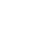… where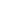is the mathematical constant that’s approximately equal to 2.71828 (AKA, Euler’s number).

That’s it! That’s all it does!

Like all of the NumPy functions, it is designed to perform this calculation with NumPy arrays and array-like structures. So essentially, the np.exp function is useful when you need to compute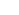for a large matrix of numbers.

So now that you know what the function does, let’s take a look at the actual syntax.

## The syntax of np.exp

The syntax of np.exp (AKA, the NumPy exponential function) is extremely simple.

Before I show it to you though, I want to make an important point.

#### A quick note

A very common convention in NumPy syntax is to give the NumPy module the alias “np“.

Technically speaking, we give NumPy this nickname when we import the NumPy module. You can do it with the code import numpy as np.

I just want to point this out, because in this tutorial (and specifically in this section about the syntax) I’m referring to NumPy as np. That will only work properly though if you import NumPy with the code import numpy as np.

I’ll show you this specifically when we look at some examples.

Ok. Let’s look at the syntax.

### NumPy exponential syntax

As I mentioned earlier, the syntax of the NumPy exponential function is extremely simple. It’s possibly one of the simplest NumPy functions.

Essentially, you call the function with the code np.exp() and then inside of the parenthesis is a parameter that enables you to provide the inputs to the function.It’s really, really simple.

Having said that though, let’s quickly talk about the parameters of np.exp.

### The parameters of np.exp

There’s really only 1 parameter that we’re going to talk about, and that’s the x parameter.

There are a few other parameters like out and where, but they are less commonly used, so we won’t cover them here.

Let’s quickly review the x parameter.

###### x (required)

The x = parameter enables you to provide the inputs to the np.exp() function.

Technically, this input will accept NumPy arrays, but also single numbers (integers or floats) or array-like objects. So you can actually use Python lists and other array-like objects as inputs to the x parameter.

Note that an input to this parameter is required. You must provide an input here.

Also, you don’t explicitly need to type x =. You can actually leave it out and just type the name of the input array inside of the parenthesis.

Ok. Now that I’ve explained the syntax, let’s take a look at some examples.

## Examples of how to use NumPy exp

Here, I’ll show you a few examples of how to use numpy.exp.

Examples:

#### Run this code first

Before you run the following examples, make sure to import NumPy properly:

import numpy as np


As I explained earlier in this tutorial, this code will import NumPy with the nickname np.

It will essentially enable you to refer to NumPy in your code as np. This is a good shorthand that makes your code a little simpler and faster to write.

Moreover, this is just the common convention, so I want you to understand it.

### Use numpy.exp with a single number

The first example will be extremely simple.

Here, instead of using the numpy.exp function on an array, we’ll just use it with a single number as an input.

np.exp(2)


OUT:

7.38905609893065


This is really, really simple. This just calculates the value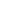.Remember: the value ofis roughly 2.71828, so when we calculate, we’re basically calculating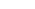= (2.71828 * 2.71828).

As calculated by NumPy,= 7.38905609893065.

### Use numpy.exp with a 1-dimensional array

Next, let’s work with a slightly more complicated example.

Here, we’re going to use a list of numbers as the input.

To be clear, this is essentially identical to using a 1-dimensional NumPy array as an input. However, I think that it’s easier to understand if we just use a Python list of numbers.

Ok, we’re basically going to use the Python list [0,1,2,3,4] as the input to the x argument.

NumPy is going to calculatefor each of these numbers.

Here’s the code:

np.exp([0,1,2,3,4])


And here’s the output:

array([ 1.,  2.71828183,  7.3890561 , 20.08553692, 54.59815003])


So what happened here?

The numpy.exp function will take each input value, [0,1,2,3,4], and apply it as the exponent to the base.Here, we’ve only used 4 values laid out in a Python list. But this will work in a similar way with a much longer list. You could have a list of hundreds, even thousands of values! The numpy.exp function will work the same.

##### Redo example with a NumPy array

Quickly, I want to re-do the above example with a proper NumPy array.

np_array_1d = np.array([0,1,2,3,4])


And let’s print it out:

print(np_array_1d)


OUT:

[0 1 2 3 4]


As you can see, this NumPy array has the exact same values as the Python list in the previous section.

Now, let’s compute the exponent with numpy.exp:

np.exp(np_array_1d)


OUT:

array([ 1. ,  2.71828183,  7.3890561 , 20.08553692, 54.59815003])


This output is essentially identical to the output created with the Python list [0,1,2,3,4].

I want to show you this to reinforce the fact that numpy.exp can operate on Python lists, NumPy arrays, and any other array-like structure.

### Use numpy.exp with a multi-dimensional array

Finally, let’s use the numpy.exp function with a 2-dimensional array.

Here, we’ll use a NumPy array. We’ll create a 2-d array using numpy.arange, which we will reshape into a 2-d form with the NumPy reshape method.

np_array_2d = np.arange(9).reshape([3,3])


Quickly, let’s print out the array, so you can see the contents:

print(np_array_2d)


OUT:

[[0 1 2]
[3 4 5]
[6 7 8]]


As you can see, this is a 2-dimensional NumPy array that contains the numbers from 0 to 8.

Now, let’s computefor each of these values using numpy.exp.

np.exp(np_array_2d)


OUT:

array([[1.00000000e+00, 2.71828183e+00, 7.38905610e+00],
[2.00855369e+01, 5.45981500e+01, 1.48413159e+02],
[4.03428793e+02, 1.09663316e+03, 2.98095799e+03]])


Once again: numpy.exp is just computingfor every value in the input array.When you give it a 2d array, the NumPy exponential function simply computesfor every input value x in the input array, and returns the result in the form of a NumPy array.

Keep in mind that np.exp works the same way for higher dimensional arrays!

## NumPy exponential FAQ

Let’s quickly cover some frequently asked questions about the NumPy exponential function.

### What does numpy.exp do?

It calculatesfor an array of input values, whereis Euler’s number: 2.71828 …

### What’s the difference between math.exp and numpy.exp?

Essentially, the math.exp() function only works on scalar values, whereas np.exp() can operate on arrays of values.

So you can use math.exp on a single number:

#THIS WORKS!
import math
math.exp(2)


But you can not use math.exp on an array-like object:

#THIS THROWS AN ERROR
import math
math.exp([1,2,3,4])


And as you saw earlier in this tutorial, the np.exp function works with both scalars and arrays.

Essentially, np.exp is more flexible than math.exp.

### WTF is Euler’s Number?

The short answer: Euler’s number (AKA,) is an interesting and important mathematical constant. It’s a number!

The value ofis roughly 2.7182818284590452353602874713527.

How exactly we arrive at this constant and what it’s good for is sort of a long answer, and beyond the scope of this blog post.

At a high level though,is a very important number in mathematics. It shows up all over the place in math, physics, engineering, economics, and just about any place that deals with exponential growth, compounded growth, and calculus.

## Check out our other NumPy tutorials

In this tutorial, you learned about the NumPy exponential function.

If you want to learn about other NumPy functions, we have quite a few other tutorials:

Those are just a few though. We also have a variety of tutorials about Matplotlib and Pandas.

## For more data science tutorials, sign up for our email list

• NumPy
• Pandas
• Base Python
• Scikit learn
• Machine learning
• Deep learning
• … and more.

We regularly publish FREE data science tutorials. We publish tutorials about NumPy, Pandas, matplotlib, and data science in Python.